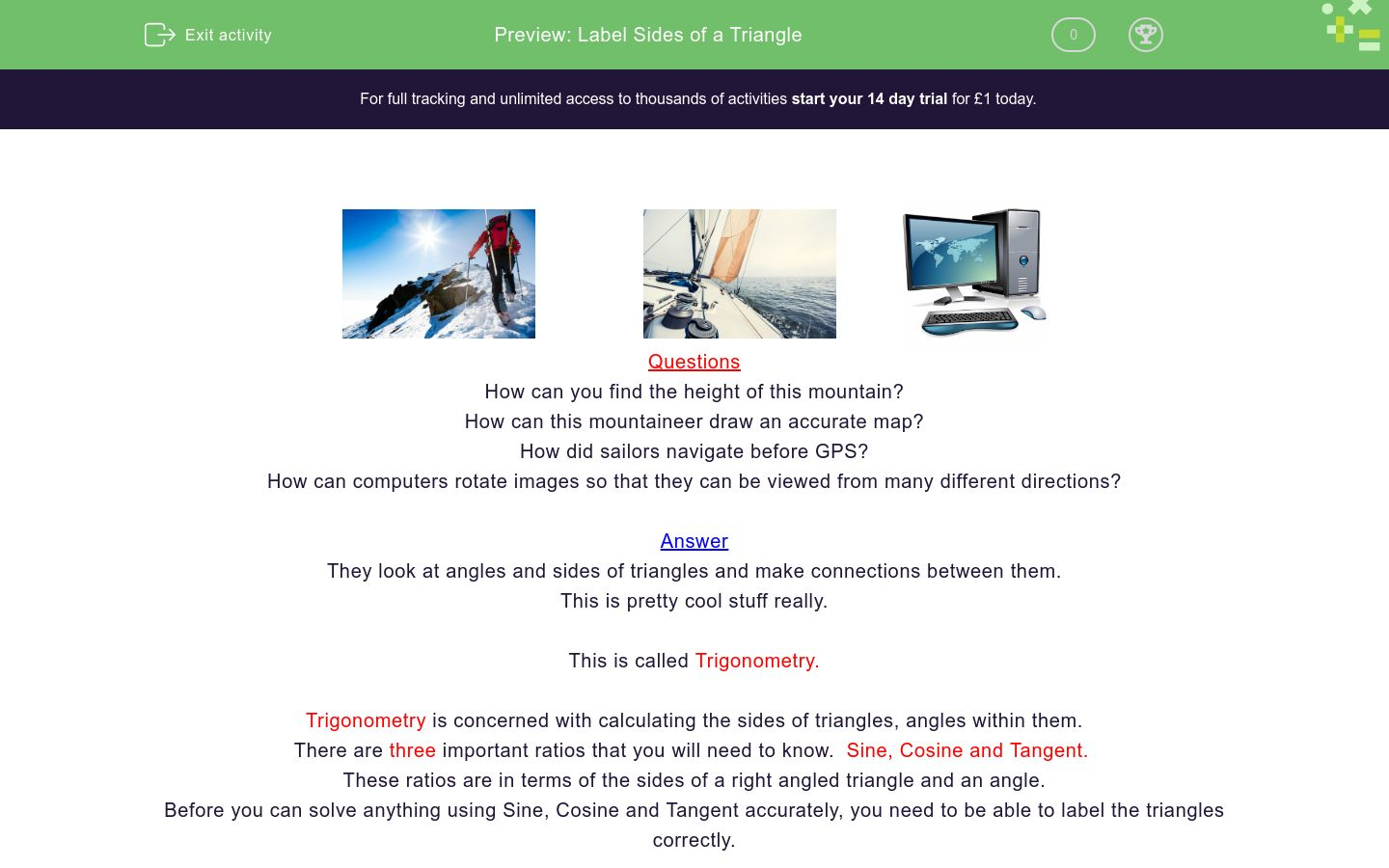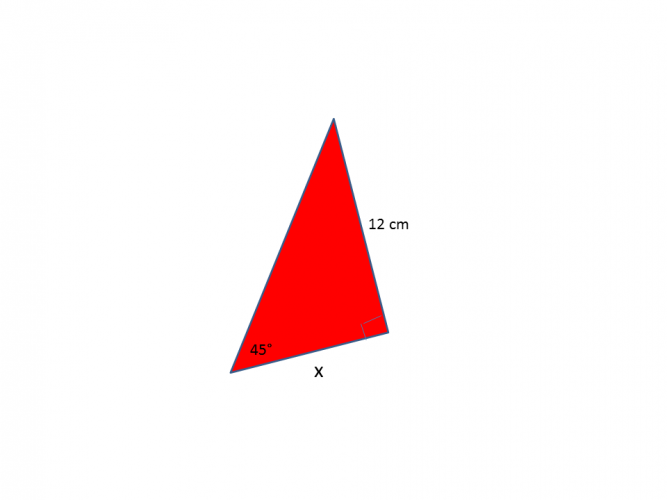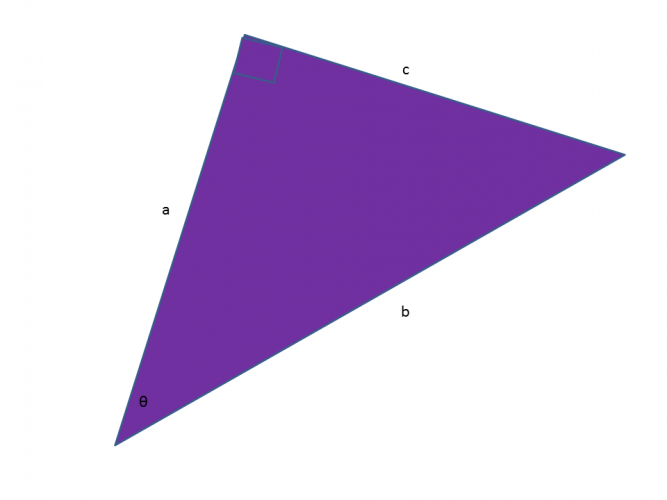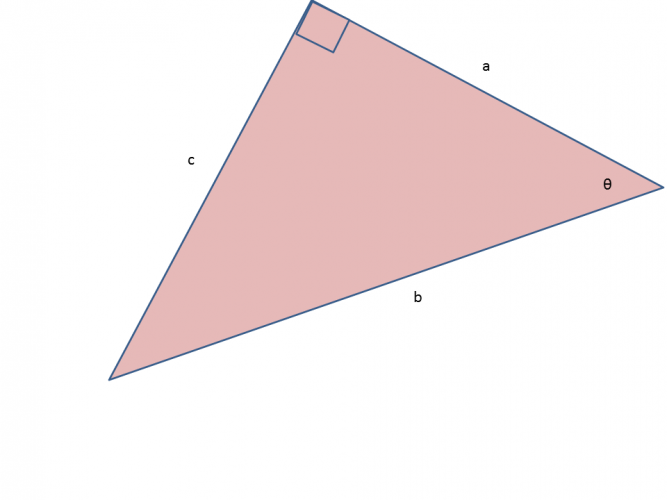# Label Sides of a Triangle

In this worksheet, students will become familiar with labelling sides of a triangle to help solve trigonometry problems.Key stage:  KS 4

GCSE Subjects:   Maths

GCSE Boards:   AQA, Eduqas, Pearson Edexcel, OCR

Curriculum topic:   Geometry and Measures, Mensuration

Curriculum subtopic:   Mensuration and Calculation, Triangle Mensuration

Difficulty level:### QUESTION 1 of 10Questions

How can you find the height of this mountain?

How can this mountaineer draw an accurate map?

How did sailors navigate before GPS?

How can computers rotate images so that they can be viewed from many different directions?

They look at angles and sides of triangles and make connections between them.

This is pretty cool stuff really.

This is called Trigonometry.

Trigonometry is concerned with calculating the sides of triangles, angles within them.

There are three important ratios that you will need to know.  Sine, Cosine and Tangent.

These ratios are in terms of the sides of a right angled triangle and an angle.

Before you can solve anything using Sine, Cosine and Tangent accurately, you need to be able to label the triangles correctly.

Things to remember

The longest side of a right angled triangle is called the hypotenuse (H)

The side opposite the angle is called the opposite side (O)

The side right next to the angle is called the adjacent side (A)Look at the diagram above.

Label the hypotenuse first, as it is the easiest to spot.

Then go to the angle and pick up the opposite side.

That just leaves the adjacent side to label.

I always do it in that order, as it can be easy to get the opposite and adjacent muddled up.

Now let's look at labelling the adjacent.When you want to find the adjacent angle, find the angle in your diagram and draw a line along the side

Let's tri...c is the hypotenuse, c is the opposite c is the adjacentWhat side is x?

Hypotenuse

OppositeWhat would you label the side 12 cm?Hypotenuse opposite adjacent side a is side b is side c isHypotenuse opposite adjacent side a is side b is side c isHow would you label angle b?

Hypotenuse

Oppositea is the hypotenuse, opposite, adjacent.## Column B

a is the
b is the
opposite
c is the
hypotenuseopposite hypotenuse adjacent a is b is c isa

b

c

• Question 1c is the hypotenuse,
c is the opposite
EDDIE SAYS
It is said that opposites attract. So just go to the angle and draw a straight line. This will take you to the opposite angle. Once you have done this a few times it becomes second nature.
• Question 2What side is x?

EDDIE SAYS
As I said if you can identify the hypotenuse and opposite first then the adjacent is the only one left. If at first you don't succeed tri, tri and tri again.
• Question 3What would you label the side 12 cm?

Hypotenuse
EDDIE SAYS
The longest side is always the hypotenuse. It is the other two sides that can trip us up at times.
• Question 4Hypotenuse opposite adjacent side a is side b is side c is
EDDIE SAYS
What... they have turned the triangle around. Okay so Hypotenuse first, draw a line away from the angle to find the opposite that just leaves the adjacent. Job done.
• Question 5EDDIE SAYS
Have you noticed that the only thing that is constant is the hypotenuse. The angle can be in one of two places, which affects where the opposite and adjacent go.
• Question 6How would you label angle b?

Hypotenuse
EDDIE SAYS
Yes of course, the longest side the hypotenuse.
• Question 7a is the hypotenuse, opposite, adjacent.
EDDIE SAYS
Are you getting the hang of this now? Adjacent is the side right next to the angle.
• Question 8## Column B

a is the
b is the
hypotenuse
c is the
opposite
EDDIE SAYS
You have to be able to label all three sides correctly when you come on to using Sin, Cos and Tan to find missing angles and sides. Keep practicing.
• Question 9opposite hypotenuse adjacent a is b is c is
EDDIE SAYS
Are you finding this a little tri ing (trying) now? Once you have this cracked your trigonometry will become much easier.
• Question 10a
EDDIE SAYS
Are you doing these in your sleep yet? Adjacent is the side right next to the angle.
---- OR ----

Sign up for a £1 trial so you can track and measure your child's progress on this activity.

### What is EdPlace?

We're your National Curriculum aligned online education content provider helping each child succeed in English, maths and science from year 1 to GCSE. With an EdPlace account you’ll be able to track and measure progress, helping each child achieve their best. We build confidence and attainment by personalising each child’s learning at a level that suits them.

Get started